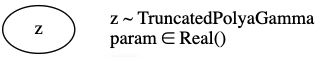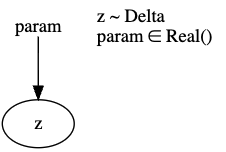# Is this graphical model expected?

I created a simple model with TruncatedPolyaGamma.

``````def model():
param = pyro.param("param", torch.ones(1))
pyro.sample("z", dist.TruncatedPolyaGamma(param))
``````

When I render the model with `pyro.render_model(model, render_distributions=True, render_params=True)` statement, I see that it looks like thisHowever, when I use another distribution, like Delta, as follows

``````def model():
param = pyro.param("param", torch.ones(1))
pyro.sample("z", dist.Delta(param))
``````

the rendered model isAs you can see, `param` is not included in the first graph. Is this the expected rendering? If it is, what is the reason for not including it in the graph?

if you read the docs you’ll see that `TruncatedPolyaGamma` is a parameter free distribution. the passed in tensor is only used to set the `dtype` and `device`